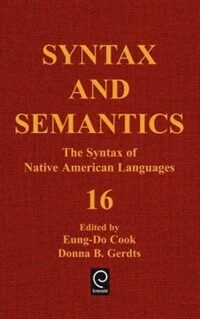> Detail View

# Detail View## Syntax and semantics. vol.16, : the syntax of native American languages

Material type
단행본
Personal Author
Cook, Eung-Do. Gerdts, Donna B.
Title Statement
Syntax and semantics. vol.16,. the syntax of native American languages / edited by Eung-Do Cook, Donna B. Gerdts.
Publication, Distribution, etc
Physical Medium
xiii, 34 p. : ill. ; 23 cm.
ISBN
0126135169 (alk. paper)
Bibliography, Etc. Note
Includes bibliographies and index.
Indians --Languages --Syntax.
 000 00768camuuu200229 a 4500 001 000000906831 005 19981216093351.0 008 830804s1984 flua b 00100 eng 010 ▼a 83017265 //r902 020 ▼a 0126135169 (alk. paper) 040 ▼a DLC ▼c DLC ▼d DLC ▼d OCoLC ▼d 244002 049 0 ▼l 452030809 ▼v 16 050 0 0 ▼a P1 ▼b .S9 vol. 16 ▼a PM123 082 0 0 ▼a 415 s ▼a 415 ▼2 19 090 ▼a 410 ▼b S992 ▼c 16 245 0 0 ▼a Syntax and semantics. ▼n vol.16,. ▼p the syntax of native American languages / ▼c edited by Eung-Do Cook, Donna B. Gerdts. 260 ▼a Orlando : ▼b Academic Press, ▼c 1984. 300 ▼a xiii, 34 p. : ▼b ill. ; ▼c 23 cm. 504 ▼a Includes bibliographies and index. 650 0 ▼a Indians ▼x Languages ▼x Syntax. 700 1 ▼a Cook, Eung-Do. 700 1 ▼a Gerdts, Donna B.

### Holdings Information

No. Location Call Number Accession No. Availability Due Date Make a Reservation Service
No. 1 Location Call Number 410 S992 16 Accession No. 452030809 Availability Available Due Date Make a Reservation Service

### Contents information

```
CONTENTS
Contributors = ⅸ
Preface = xi
Themes and Absolutives ： Some Semantic Rules in Tzotzil / JUDITH AlSSEN = 1
1. Theme and Absolutive = 1
2. Assumptions = 2
3. Tzotzil = 3
4. Quantification = 4
5. Themes, Initial Absolutives, and Antipassive = 10
6. The Distinctness of Initial Absolutives and Themes = 15
7. Conclusion = 19
References = 19
Kwakwala Syntax and the Government­Binding Theory / STEPHEN R. ANDERSON = 21
1. A Sketch of Kwakwala Structure = 24
2. Special Properties of Kwakwala Subjects = 28
3. Distinguishing Subjects from Nonsubjects = 43
4. Subjects versus Nonsubjects in Kwakwala = 48
5. Conclusion = 66
References = 74
Moving Interrogatives without an Initial + WH Node in Tup\$\$\acute i\$\$ / FRANK ROBERTS BRANDON ; LUCY FERREIRA SEKI = 77
1. Introduction = 77
2. Interrogative Sentences in Tup\$\$\acute i\$\$ = 81
3. Q­Substitution and Tup\$\$\acute i\$\$ = 87
4. Conclusions = 99
References = 102
Clause Reduction in Ancash Quechua / PETER COLE = 105
1. Introduction = 105
2. Ancash = 106
3. The Distribution of Putative Object­to­Object Raising in Ancash = 108
4. Properties of Clause Reduction versus Object­to­Object Raising = 110
5. Validation = 111
6. Object­Verb Agreement = 113
7. Word Order = 115
8. Conclusions = 116
Appendix = 117
References = 120
Choctaw Switch­Reference and Levels of Syntactic Representation / WILLIAM D. DAVIES = 123
1. Introduction = 123
2. Switch­Reference Phenomena = 124
3. Some Basic Notions of Relational Grammar = 126
4. Inversion in Choctaw = 128
5. The Inversion Structure = 136
6. Switch­Reference in Choctaw = 139
7. Conclusion = 145
References = 146
An Expression of Coreference in Bella Coola / PHILIP W. DAVIS ; ROSS SAUNDERS = 149
1. Introduction = 149
2. A Rule of Pronominalization = 152
3. The Anaphoric Pattern = 158
4. The Cataphoric Pattern = 162
5. Conclusion = 164
References = 167
A Relational Analysis of Halkomelem Causals / DONNA B. GERDTS = 169
1. Introduction = 169
2. Outline of Relational Grammar = 171
3. Some Basic Rules of Halkomelem = 176
4. Causals in Halkomelem = 181
5. Causal Constructions and the Unaccusative Hypothesis = 189
6. Passives of Caus­2 Constructions and the 1­AEX = 199
7. Conclusion = 202
References = 203
Raising to Subject in Moose Cree ： A Problem for Subjacency / DEBORAH JAMES = 205
1. Background Data = 205
2. Evidence for the Existence of Raising to Subject = 207
3. Implications for Linguistic Theory = 210
References = 213
Empty Categories, Rules of Grammar, and Kwakwala Complementation / ROBERT D. LEVINE = 215
1. Introduction = 215
2. Alternative Analyses = 217
3. Anaphora as a Logical Operation = 238
References = 245
Switch­Reference and Subject Raising in Seri / STEPHEN A. MARLETT = 247
1. Introduction = 247
2. Basic Switch­Reference Facts = 248
3. Basic Subject Raising Facts = 250
4. The Notion "First Subject" = 263
5. Conclusions = 266
References = 267
Floating Quantifiers in Pima / PAMELA MUNRO = 269
1. Pima: An Introduction = 269
2. Quantifier Float in Pima = 272
3. Interpretation of Pima Floated Quantifiers = 276
4. Combinations of Floated Quantifiers = 283
5. Floating Quantifiers and Pima Syntactic Structure References = 286
On the Nonergativity and Intransitivity of Relative Clauses in Labrador Inuttut / LAWRENCE R. SMITH = 289
1. Introduction = 289
2. Background and Theoretical Relevance = 291
3. An Initial Example of Relativization = 293
4. An Array of Relative Clause Structures = 295
5. An Apparent Generalization about Relative Clauses = 298
6. Transitivity in Relative Clauses = 299
7. Relativization into the Subject of the Transitive Relative Clause and Detransitivization = 302
8. Relativization into the Object of the Relative Clause = 306
9. A Reanalysis of Putative Transitive Relative Clauses = 308
10. Conclusion = 312
References = 314
Index = 317
Contents of Previous Volumes = 325

```

### New Arrivals Books in Related Fields

#### 야콥슨-레비스트로스 서한집

Jakobson, Roman (2023)

#### 数の値打ち : グローバル情報化時代に日本文学を読む

Long, Hoyt J (2023)

신동일 (2023)

김기덕 (2023)

#### Affaires de style

Cafiero, Florian (2022)